### Home > AC > Chapter 14 > Lesson 14.2.1.7 > Problem2-102

2-102.
1. For the function g(x) = x3 + x2 − 6x , find the value of each expression below. Homework Help ✎

1. g(1)

2. g(−1)

3. g(−2)

4. g(10)

5. Find at least one value of x for which g(x) = 0.

6. If f(x) = x2x + 3, find g(x) − f(x).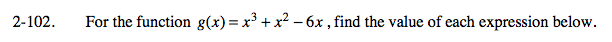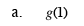Substitute 1 for x in the given equation and then evaluate.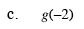8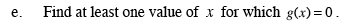Let g(x) = 0 in the original equation and then solve for x.

x = −3, 0, 2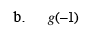6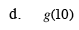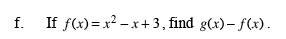g(x) − f(x) = (x3 + x2 − 6x) − (x2x + 3)